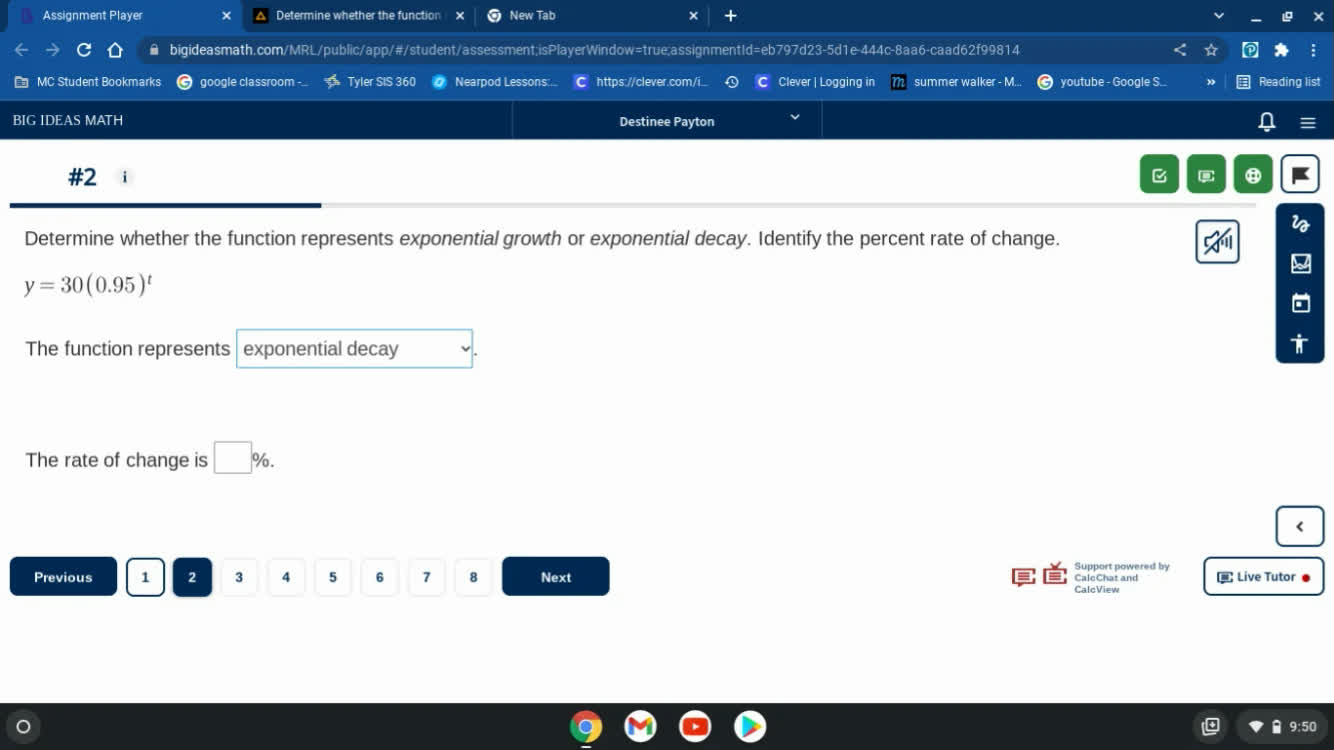# Get help with exponential growth and decay

Recent questions in Exponential growth and decayjamstavajo 2022-09-03

2022-05-18

2022-04-21

### Scientists measure a bacteria population and find that it is 2,700. Three days later, they find that the population has doubled. Write a function f(d) that could describe the bacteria population d days after the scientists first measured it, assuming it grows exponentially?Samuel Herrera Liberato 2022-03-30

2022-03-28

2022-03-23

### At the beginning of the year, there are 7650 individuals in a population of beavers whose per capita rate of for the year is 0.18. What is its population growth rate at the end of the year?MMDCCVIII446 2022-02-14

### Determine whether the function represents exponential growth or exponential decay. Identify the percent rate of change. y=30(0.95)^tgravaviwoa 2022-02-13

2022-02-03

### # State whether the equation represents exponential growth, exponential decay,f(x)=14⋅12xMaiclubk 2021-09-27

### Without graphing, determine whether the function $y=0.3\left(1.25{\right)}^{x}$ represents exponential growth or decay. State how you made the determination.Alyce Wilkinson 2021-09-21

### Solve the equation. Round your answers to the nearest hundredth. ${8}^{x}=12$Ramsey 2021-09-20

### Solve the equation. Round your answers to the nearest hundredth. ${2}^{x}=100$Trent Carpenter 2021-09-18

### Determine whether each function is an example of exponential growth or decay. Then find the y-intercept. $y=0.6\left(1/10{\right)}^{x}$BenoguigoliB 2021-07-31

### Determine whether the function represents exponential growth or exponential decay. Identify the percent rate of change. Then graph the function. $f\left(x\right)={\left(0.2\right)}^{x}$boitshupoO 2021-07-05

### Determine whether the function represents exponential growth or exponential decay. Identify the percent rate of change. $f\left(t\right)=6\left(0.84{\right)}^{t}$boitshupoO 2021-07-04

### Without graphing, determine whether the function $y=0.3\left(1.25\right)x$ represents exponential growth or decay. State how you made the determination.preprekomW 2021-07-04

### Determine whether the function $y=156{\left(0.825\right)}^{t}$ represents exponential growth, exponential decay, or neither. ExplainAlbarellak 2021-06-27

### Identify each of the following functions as exponential growth or decay. Then give the rate of growth or decay as a percent. $y=a{\left(\frac{1}{2}\right)}^{t}$UkusakazaL 2021-06-23

### Determine whether each function is an example of exponential growth or decay. Then, find the y-intercept. $y=25/7\left(7/5{\right)}^{x}$Cabiolab 2021-06-22

### Find the multiplier for the rate of exponential growth or decay. 0.4% growth _______

When exponential growth is defined, it means that the base of our exponential is bigger than one, meaning that those particular numbers increase. These are the exponential growth and decay problems where the value is taken first in relation to the function. If it doesn’t sound familiar, look into the exponential growth and decay questions that are provided. These will help you find the answers as you are struggling with your exponential growth and decay homework. Of course, in some cases, you will need a particular exponential growth and decay solver, which is why exploring these sample solutions will help.